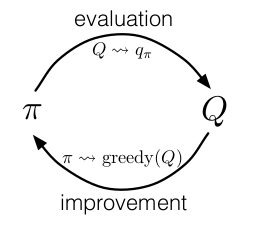[ 强化学习  ]

强化学习基础 4：蒙特卡洛方法

一、蒙特卡洛预测

方法

\begin{algorithm}
\caption{First-visit MC prediction}
\begin{algorithmic}
\INPUT $\pi$, policy to be evaluated
\OUTPUT $V\approx v_\pi$

\STATE initialize $V(s)\in\mathbb{R}$, arbitrarily, for all $s\in\mathcal{S}$
\STATE $Returns(s)\gets$ an empty list, for all $s\in\mathcal{S}$
\REPEAT
\STATE Generate a trajectory following $\pi:S_0,A_0,R_1,S_1,A_1,\dots,S_{T-1},A_{T-1},R_T$
\STATE $G \gets 0$
\FORALL{$t=T-1,T-2,\dots,0$}
\STATE $G\gets\gamma G+R_{t+1}$
\IF{\NOT $S_t$ appears in $S_0,S_1,\dots,S_{t-1}$}
\STATE Append $G$ to $Returns(S_t)$
\STATE $V(S_t)\gets$ average($Returns(S_t)$)
\ENDIF
\ENDFOR
\UNTIL{$V$ is stable}
\RETURN $V$
\end{algorithmic}
\end{algorithm}

与 DP 对比

MC 方法可以从实际经验或模拟经验中学习，不需要环境的先验知识，因此适用范围更广；MC 仅仅计算采样的部分，不需要对所有状态空间都计算，因此计算效率更高（例如，MC 可以仅仅计算某个你关心的状态的 value，其他状态都可以不计算。毕竟 MC 不是 bootstrapping 方法，不需要其他状态的估计值）。

三、蒙特卡洛控制1. 能够观察到无穷个 episode （轮数趋于无穷）
2. exploring starts 假设

\begin{algorithm}
\caption{Monte Carlo ES (Exploring Starts)}
\begin{algorithmic}
\OUTPUT $\pi\approx\pi_\ast$

\STATE Initialize $\pi(s)\in\mathcal{A}(s)$ (arbitrarily), for all $s\in\mathcal{S}$
\STATE Initialize $Q(s,a)\in\mathbb{R}$ (arbitrarily), for all $s\in\mathcal{S},a\in\mathcal{A}$
\STATE Initialize $Returns(s,a)\gets$ emtpy list, for all $s\in\mathcal{S},a\in\mathcal{A}$
\REPEAT
\STATE Choose $S_0\in\mathcal{S},A_0\in\mathcal{A}(S_0)$ randomly such that all pairs have probability $>0$
\STATE Generate a trajectory following $\pi:S_0,A_0,R_1,S_1,A_1,\dots,S_{T-1},A_{T-1},R_T$
\STATE $G \gets 0$
\FORALL{$t=T-1,T-2,\dots,0$}
\STATE $G\gets\gamma G+R_{t+1}$
\IF{\NOT $S_t,A_t$ appears in $S_0,A_0,S_1,A_1,\dots,S_{t-1},A_{t-1}$}
\STATE Append $G$ to $Returns(S_t,A_t)$
\STATE $Q(S_t,A_t)\gets$ average($Returns(S_t,A_t)$)
\STATE $\pi(S_t)\gets\argmax_aQ(S_t,a)$
\ENDIF
\ENDFOR
\UNTIL{$\pi$ is stable}
\RETURN $\pi$
\end{algorithmic}
\end{algorithm}

四、无 Exploring Starts 假设的蒙特卡洛控制

$\varepsilon$-greedy 策略是 $\varepsilon$-soft 策略的一种实例（$\varepsilon$-soft 策略定义为：对某个 $\varepsilon>0$ 和任意状态和动作，有 $\pi(a\vert s)\ge\frac{\varepsilon}{\vert \mathcal{A}(s)\vert}$），在所有 $\varepsilon$-soft 策略中，$\varepsilon$-greedy 策略是最接近贪婪策略的。

\begin{algorithm}
\caption{On-policy first-visit MC control (for $\varepsilon$-soft policies)}
\begin{algorithmic}
\Input $\varepsilon>0$
\Output $\pi\approx\pi_\ast$

\STATE Initialize $\pi(s)\in\mathcal{A}(s)$ (arbitrarily), for all $s\in\mathcal{S}$
\STATE Initialize $Q(s,a)\in\mathbb{R}$ (arbitrarily), for all $s\in\mathcal{S},a\in\mathcal{A}$
\STATE Initialize $Returns(s,a)\gets$ emtpy list, for all $s\in\mathcal{S},a\in\mathcal{A}$
\REPEAT
\STATE Choose $S_0\in\mathcal{S},A_0\in\mathcal{A}(S_0)$ randomly such that all pairs have probability $>0$
\STATE Generate a trajectory following $\pi:S_0,A_0,R_1,S_1,A_1,\dots,S_{T-1},A_{T-1},R_T$
\STATE $G \gets 0$
\FORALL{$t=T-1,T-2,\dots,0$}
\STATE $G\gets\gamma G+R_{t+1}$
\IF{\NOT $S_t,A_t$ appears in $S_0,A_0,S_1,A_1,\dots,S_{t-1},A_{t-1}$}
\STATE Append $G$ to $Returns(S_t,A_t)$
\STATE $Q(S_t,A_t)\gets$ average($Returns(S_t,A_t)$)
\STATE $A^\ast\gets\argmax_aQ(S_t,a)$
\FORALL{$a\in\mathcal{A}(S_t)$}
\STATE $\pi(a\vert S_t)\gets\begin{cases}1-\varepsilon+\frac{\varepsilon}{\vert \mathcal{A}(s)\vert}\quad&\text{if }a=A^\ast\\\frac{\varepsilon}{\vert \mathcal{A}(s)\vert}\quad&\text{if }a\ne A^\ast\end{cases}$
\ENDFOR
\ENDIF
\ENDFOR
\UNTIL{$\pi$ is stable}
\RETURN $\pi$
\end{algorithmic}
\end{algorithm}